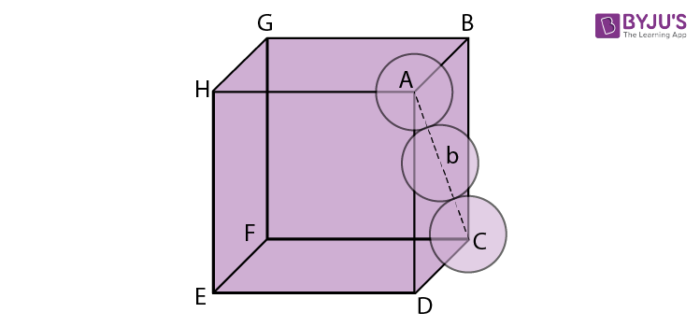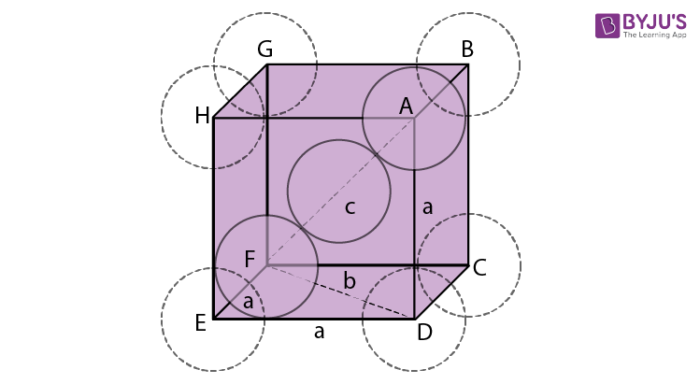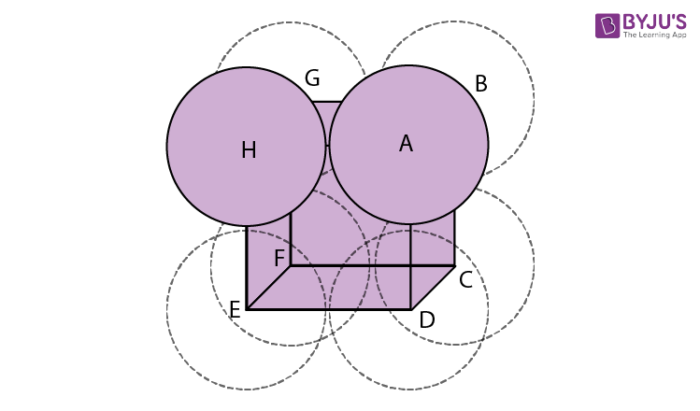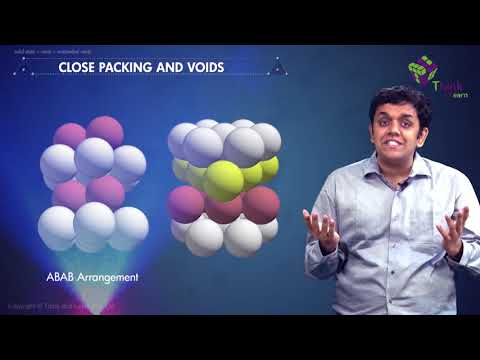# Packing Efficiency Of A Unit Cell

A crystal lattice is made up of a very large number of unit cells where every lattice point is occupied by one constituent particle. The unit cell can be seen as a three dimension structure containing one or more atoms. We always observe some void spaces in the unit cell irrespective of the type of packing. The Percentage of spaces filled by the particles in the unit cell is known as the packing fraction of the unit cell. In this section, we shall learn about packing efficiency. The packing fraction of different types of packing in unit cells is calculated below:

## Packing efficiency in face centered unit cell and Cubic close packing structure:

Hexagonal close packing (hcp) and cubic close packing (ccp) have the same packing efficiency. Let us take a unit cell of edge length “a”. Length of face diagonal, b can be calculated with the help of Pythagoras theorem,$$\begin{array}{l} b^{2} = a^{2} + a^{2}\end{array}$$

The radius of the sphere is r
The face diagonal (b) = r + 2r + r = 4r

$$\begin{array}{l} \therefore (4r)^{2} = a^{2} + a^{2}\end{array}$$

$$\begin{array}{l} \Rightarrow (4r)^{2} = 2a^{2}\end{array}$$

$$\begin{array}{l} \Rightarrow a = \sqrt{\frac{16r^{2}}{2}}\end{array}$$

$$\begin{array}{l} \Rightarrow a = \sqrt{8} r\end{array}$$

Volume of the cube = a3 =

$$\begin{array}{l}(\sqrt{8} r)^{3}\end{array}$$

Volume of the sphere = (4/3) π r3

No. of Sphere present in one FCC unit cell =4

The volume of the sphere = 4 x (4/3) π r3

$$\begin{array}{l} The\ Packing\ efficiency =\frac{Total\ volume\ of\ sphere}{volume\ of\ cube}\times 100\end{array}$$

$$\begin{array}{l} =\frac{\frac{16}{3}\pi r^{3}}{8\sqrt{8}r^{3}}\times 100\end{array}$$

= 74%

## Packing efficiency in body centered cubic unit cell:

In body centered cubic unit cell, one atom is located at the body center apart from the corners of the cube. Let us take a unit cell of edge length “a”. Length of body diagonal, c can be calculated with help of Pythagoras theorem,$$\begin{array}{l} c^2~=~ a^2~ + ~b^2 \end{array}$$

Where b is the length of face diagonal, thus b

$$\begin{array}{l}= \sqrt{2}~a\end{array}$$
$$\begin{array}{l} c^2~=~ 3a^2\end{array}$$
$$\begin{array}{l} c = \sqrt{3} a\end{array}$$

From the figure, radius of the sphere, r = 1/4 × length of body diagonal, c

$$\begin{array}{l}r = \frac {c}{4}\end{array}$$
=
$$\begin{array}{l} \frac{\sqrt{3}}{4}~a\end{array}$$
$$\begin{array}{l} a = \frac {4}{\sqrt{3}} r\end{array}$$

In body centered cubic structures, each unit cell has two atoms,

$$\begin{array}{l}Packing\ efficiency = \frac{volume~ occupied~ by~ two~ spheres~ in~ unit~ cell}{Total~ volume~ of~ unit ~cell} × 100\end{array}$$
$$\begin{array}{l}= \frac {2~×~\left( \frac 43 \right) \pi r^3~×~100}{( \frac {4}{\sqrt{3}})^3}\end{array}$$

= 68%

## Packing Efficiency of Diamond

In the structure of diamond, C atom is present at all corners, all face centres and 50 % tetrahedral voids.

$$\begin{array}{l}Bond\ length\ i.e\ distance\ between\ 2\ nearest\ C\ atom = \frac{\sqrt{3}a}{8}\end{array}$$

Radius of C atom = rc

$$\begin{array}{l}rc = \frac{\sqrt{3}a}{8}\end{array}$$

Packing efficiency of diamond is 34%

– wherein

Number of C atoms per unit cell = 8

a = edge length of unit cell

## Packing efficiency in simple cubic unit cell:

In a simple cubic unit cell, atoms are located at the corners of the cube. Let us take a unit cell of edge length “a”. Radius of the atom can be given as,$$\begin{array}{l}r = \frac a2 \end{array}$$

=> a = 2r

In simple cubic structures, each unit cell has only one atom,

$$\begin{array}{l}Packing\ efficiency = \frac{volume~ occupied~ by~ one~ atom}{Total~ volume~ of~ unit ~cell} × 100\end{array}$$
$$\begin{array}{l}= \frac {\left( \frac 43 \right) \pi r^3~×~100}{( 2 r)^3} \end{array}$$

= 52.4%

For detailed discussion on calculation of packing efficiency, download BYJU’S – the learning app.

## Recommended Videos

### Octahedral Voids in HCP### 1. What is packing efficiency?

The percentage of the total space which is occupied by the particles in a certain packing is known as packing efficiency. The constituent particles i.e. atoms, ions or molecules are closely packed in the crystal lattice. They can do so either by cubic close packing(ccp) or by hexagonal close packing(hcp). In both the cases, a number of free spaces or voids are left i.e, the total space is not occupied.

### 2. What is the packing efficiency in HCP and CCP structure?

The Packing efficiency of Hexagonal close packing (hcp) and cubic close packing (ccp) is 74%.

### 3. Is CCP and FCC the same?

The cubic closed packing is CCP, FCC is cubic structures entered for the face. When we put the atoms in the octahedral void, the packing is of the form of ABCABC, so it is known as CCP, while the unit cell is FCC. These are two different names for the same lattice.

### 4. What is the packing efficiency in BCC?

The packing efficiency of body-centred cubic unit cell (BCC) is 68%.

### 5. What is the packing efficiency in SCC?

The packing efficiency of simple cubic unit cell (SCC) is 52.4%.

Test Your Knowledge On Unit Cell Packing Efficiency!